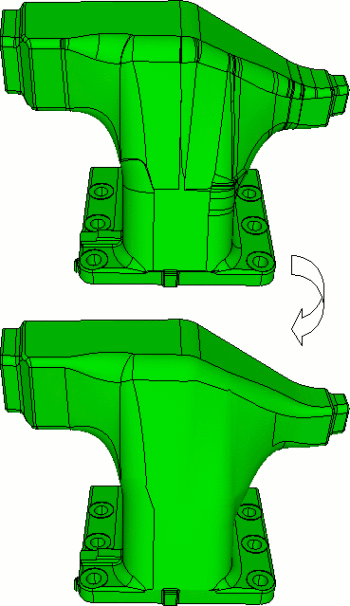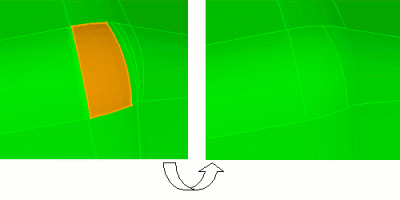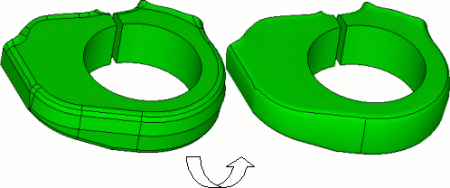# Simplify Geometry

Simplifying topology by compositing individually selected surfaces is often a tedious and time-consuming task. The simplify command addresses the tedium by automatically compositing surfaces and curves based on selected criteria between neighboring entities. Figure 1 shows a typical example of simplify command usage (‘simplify volume 1 angle 15’).Figure 1. Typical Simplify command usage

The command syntax and discussion items are shown below.

Simplify {Volume|Surface|Curve} <range> [Angle< value >] [Respect {Surface <id_range> | Curve <id_range> | Vertex <id_range>| Imprint | Fillet}] [Local_Normals] [Preview]

## Feature Angle

Feature angle is defined as the angle between the average facet normals of two neighboring surfaces. If the angle is less than the specified angle then the two surfaces are composited together (assuming any other specified criteria are met). Feature angle is always used as criteria and if an angle is not specified the value is set to 15 degrees.

## Automatically Compositing Curves

The simplify command can also be used to automatically composite curves using an angle tolerance. Curves will be composited together only if they are explicitly specified in this command, and not as the result of two surfaces being composited.

## Respecting Vertices, Curves and Surfaces

Surfaces, curves, and vertices can be specified to prevent geometry features from automatically being composited. Figure 2 show an example of respecting a surface (‘simplify vol 1 angle 15 respect surf 289’).Figure 2 Respecting a surface

For complex geometries, it is often useful to preview the simplify command and then add any respected geometry to the command respect lists.

## Respecting Imprints

Curves created by imprints can automatically be respected by the simplify command. Figure 3 shows an example of geometry with split fillets.Figure 3 Respecting imprint geometry

Notice that in the split curves are respected by the Simplify command (‘simplify vol 1 angle 40 respect imprint’).

## Using Local Normals

By default the command will compare the average normal of two adjacent surfaces to determine whether they should be composited. By issuing the local_normal option, the test will be modifed slightly. The modified test will compare the maximum difference between normals along the shared curve(s) for the two surfaces.Figure 4. Comparison of surface normals using the average surface normal method (on the left) and local normal method (on the right).

## Other Options

The preview option shows what curves are respected without compositing any surfaces. It should also be pointed out that multiple respect specifications can be chained together. For example:

Simplify volume 1 angle 15 respect curve 1 respect imprint respect fillet preview